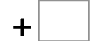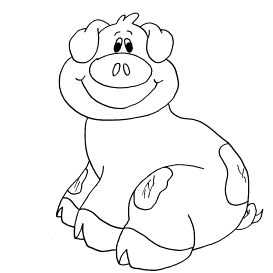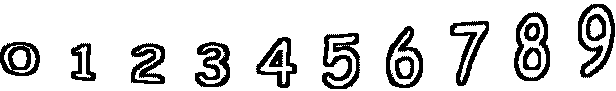71= 110   14= 5574= 151   49= 101   80= 136   52= 116 89= 184   31= 72   82= 179   14= 71